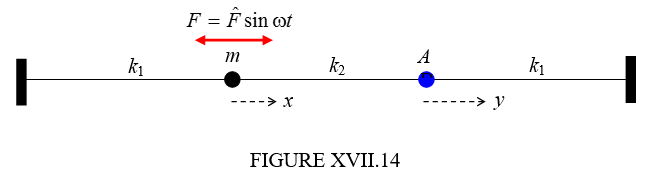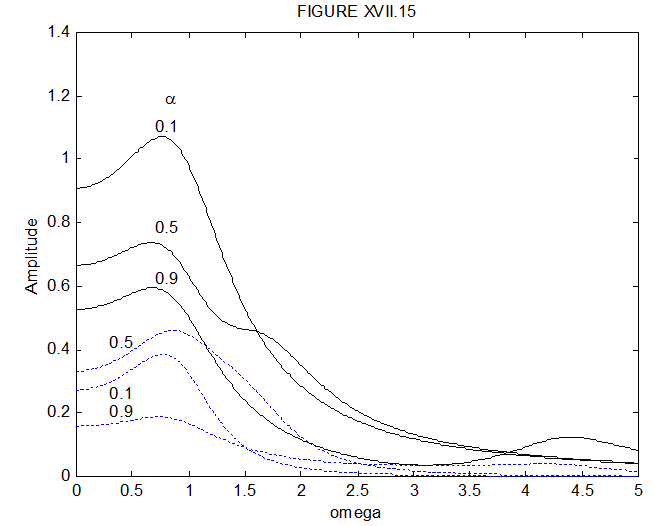$$\require{cancel}$$

# 17.13: A Damped Driven System

•• Contributed by Jeremy Tatum
• Emeritus Professor (Physics & Astronomy) at University of Victoria

I’ll leave the reader to add some damping to the system described in Section 17.12. Let us here try it with the system described in Section 17.7. We’ll apply a periodic force to the left hand mass, and we’ll suppose that the damping constant for each mass is $$\gamma=\frac{b}{m}$$. We could write the periodic force as $$F=\hat{F}\sin\omega t$$, but the algebra will be easier if we write it as $$F=\hat{F}e^{i\omega t}$$. If the initial condition is such that $$F=0$$ when $$t=0$$, then we choose just the imaginary part of this in subsequent expressions.

The equations of motion are

$$m\ddot{x}$$ = - the damping force $$b\dot{x}$$

- the tension in the left hand spring $$k_{1}x$$

+ the force $$F$$

+ the tension in the middle spring $$k_{2}(y-x)$$

(this last is a thrust whenever $$y<x$$)

and

$$m\ddot{y}$$ - the damping force $$b\dot{y}$$

- the thrust in the right hand spring $$k_{1}y$$

- the tension in the middle spring $$k_{2}(y-x)$$That is,

$m\ddot{x}\ +\ b\dot{x}\ +\ (k_{1}+k_{2})x\ -\ k_{2}y\ =\ \hat{F}e^{i\omega t} \label{17.13.1}$

and

$m\ddot{y}\ +\ b\dot{y}\ +\ (k_{1}+k_{2})y\ -\ k_{2}y\ =\ 0. \label{17.13.2}$

For the steady-state motion, seek solutions of the form

$$\ddot{x}=-\omega^{2}x$$, $$\ddot{y}=-\omega^{2} y$$, so that $$\dot{x}=i\omega x$$ and $$\dot{y}=i\omega y$$.

The equations then become

$(k_{1}\ +\ k_{2}\ -m\omega^{2}\ +\ ib\omega)x\ -\ k_{2}y\ =\ \hat{F}e^{i\omega t} \label{17.13.3}$

and

$-\ k_{2}x\ +\ (k_{1}\ +\ k_{1}\ -m\omega^{2}\ +\ ib\omega)y\ =\ 0. \label{17.13.4}$

There is now a little algebra to be carried out. Solve these equations for $$x$$ and $$y$$, and when, in doing so, there is a complex number in the denominator, multiply top and bottom by the conjugate in the usual way, so as to get $$x$$ and $$y$$ in the forms $$x'\ +\ ix''$$ and $$y'\ +\ iy''$$. Then find expressions for the amplitudes $$\hat{x}$$ and $$\hat{y}$$. After some algebra, the amount of which depends on one’s skill, experience and luck (it is not always obvious how to gather terms in the most economical way, and you need some luck in this) you eventually get, for the amplitudes of the motion

$\hat{x}^{2}=\frac{((k_{1}\ +\ k_{2}\ -m\omega^{2})\ +\ b^{2}\omega^{2})\hat{F}^{2}}{((k_{1}-m\omega^{2})^{2}+\ b^{2}\omega^{2})((k_{1}+2k_{2}-m\omega^{2})^{2}+\ b^{2}\omega^{2})} \label{17.13.5}$

and

$\hat{y}^{2}=\frac{k_{2}^{2}\hat{F}^{2}}{((k_{1}-m\omega^{2})^{2}+\ b^{2}\omega^{2})((k_{1}+2k_{2}-m\omega^{2})^{2}+\ b^{2}\omega^{2})}. \label{17.13.6}$

There are many variables in these expressions, but in order to see qualitatively what the steady state motion is like, I’m going to put $$\hat{F}$$, $$m$$ and $$k_{1}=1$$. I think if I also put $$b=1$$, this will give light damping in the sense described in Chapter 11. As for $$k_{2}$$, I am going to introduce a coupling coefficient $$\alpha$$ defined by $$\alpha\ =\ \frac{k_{2}}{k_{1}+k_{2}}$$ or $$k_{2}\ =\ \left(\frac{\alpha}{1-\alpha}\right)k_{1}$$. This coupling constant will be close to zero if the middle spring is very weak, and 1 if the middle connector is a rigid rod. The equations now become

$\hat{x}^{2}=\frac{((\frac{1}{1-\alpha}\ -\omega^{2})^{2}\ +\ \omega^{2})}{((1-\omega^{2})^{2}+\ \omega^{2})(\frac{1+\alpha}{1-\alpha}-\omega^{2})^{2}+\ \omega^{2})}. \label{17.13.7}$

and

$\hat{y}^{2}=\frac{\frac{\alpha}{(1-\alpha)}}{((1-\omega^{2})^{2}+\ \omega^{2})(\frac{1+\alpha}{1-\alpha}-\omega^{2})^{2}+\ \omega^{2})} \label{17.13.8}$

For computational efficiency you might want to rewrite these equations a little. For example you could write $$(1-\omega^{2})^{2}\ +\ \omega^{2}$$ as $$1\ -\ \Omega(1-\Omega)$$, where $$\Omega\ =\ \omega^{2}$$. In any case, figure XVII.15 shows the amplitudes of the motions of the two masses as a function of frequency, for $$\alpha\ =\ 0.1,\ 0.5$$ and $$0.9$$. The continuous black curves are for the left hand mass; the dashed blue curve is for the right hand mass.